Start typing, then use the up and down arrows to select an option from the list.# Analytical Chemistry

Learn the toughest concepts covered in your Analytical Chemistry class with step-by-step video tutorials and practice problems.

EDTA Titrations

## EDTA

1

#### concept

EDTA8m
Play a video:

EDTA represents a hexaprotic compound that possesses 7 different forms based on the pH of the solution.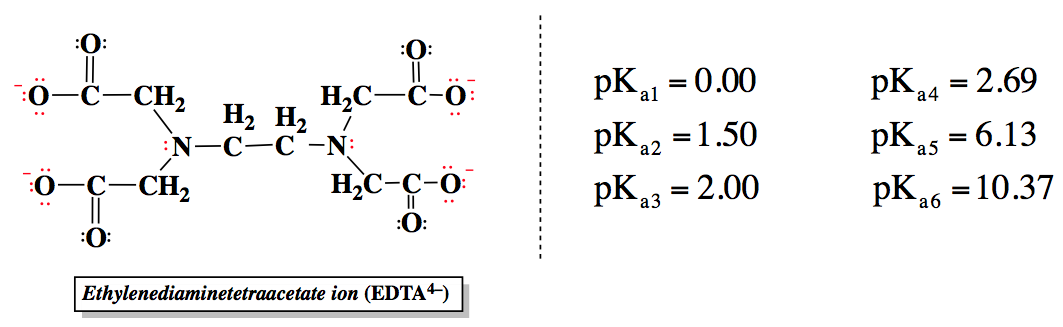The basic form of EDTA, Y4-, is the predominate form at higher pH values, while the acidic form, H6Y2+, predominates at lower pH values.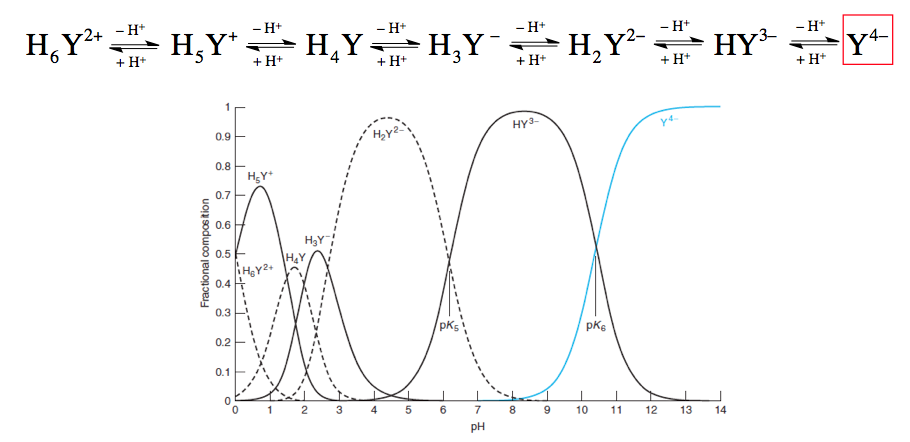The fraction of basic form present in solution can be determined by either given concentrations or by the pH of the solution.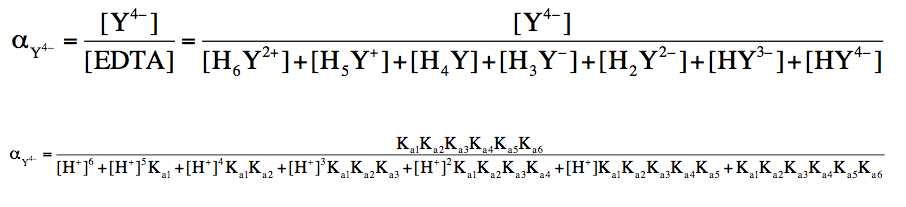## EDTA Calculations

2

#### example

EDTA Calculations 13m
Play a video:
So here it states the formal concentration of E. D. T. A. Is 1.50 millimeter. Now we're asked, what is the concentration of the basic form at a ph of 5.0. Alright so we need to determine what the concentration of our basic form will be at this given ph and here we have the overall concentration of E. D. T. A. Out of this total concentration, a portion of it belongs to the basic form. So we're gonna say that the concentration of my basic form equals the initial concentration of E. D. T. As a whole times the fraction of E. D. T. A. That exists in the basic form which we designate as alpha. So here we're gonna change our concentration to molar itty, you can keep it in millimeter but I'll just change it to polarity. So remember here that one million moller is equal to 10 to the -3 molar. So here we have 1.50 times 10 to the -3 molar. And here we're dealing with a ph of five. So what we have here are all our ph values set to a temperature of 25 degrees Celsius and an ionic strength of 250.10 Moeller. Remember what do we notice as the ph is increasing as we go from 0 to 14. Look at the fraction that exists in the basic form, we can see that it's increasing and this makes sense because on the previous page we saw that chart with the waves and the lines and we saw that as the ph increased, we got more and more of our basic form. Taking up the um the predominant form of the E. D. T. A solution. And that's all this chart here is telling us as the ph is increasing a greater percentage or a greater fraction of my total E. D. T. A solution exists in the basic form. And what this is telling me is that once I get to phs of 13 and 14 it's so basic that 100% of my total E. D. T. A solution is in the basic form. So all we do now is we just have to look up five for ph and here is my alpha for the basic form at that particular ph so we're just gonna plug that value in. So when we multiply that we get 4.35 times 10 to the -10 moller. So what that answer is telling me, it's telling me that the total amount of E. D. T. A. With all seven forms together totals 1.50 times 10 to negative three molar out of that amount. This is how much of of it exists in the basic form at that ph of five. So just remember this goes in hand with the graph that we saw on the previous page with the waves as the ph is increasing the basic form becomes more and more a predominant part of my overall solution. Now that we've seen this, take a look at example two once you attempted. Or even if you don't attempt it, don't worry, just come back and see how I approach example, too.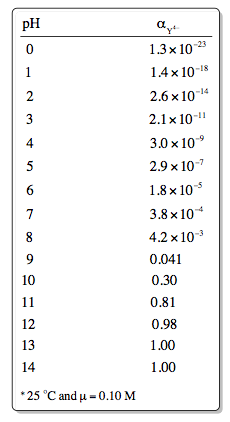3

#### example

EDTA Calculations 17m
Play a video:
4
Problem

Determine the pH where αY4– equals 0.20.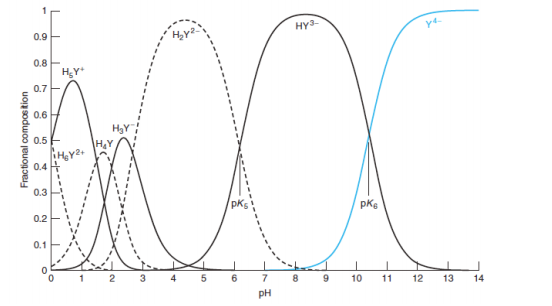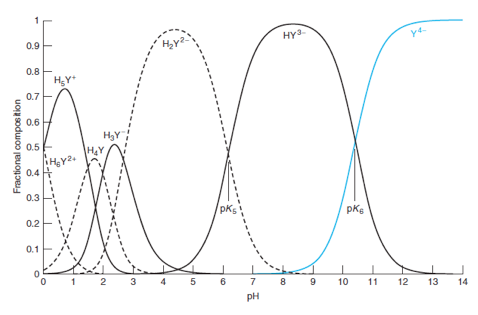## EDTA Complexes

5

#### concept

EDTA Complexes

The video is coming soon
So with E. D. T. A complexes comes the discussion of our formation or stability constants. So formation or stability constants represents the equilibrium constant for the reaction between the ligand and a medal here. Our formation or stability constant as shown by this variable. Now the lingering question that we're dealing with is E. D. T. A. Now the general formula is our metal ion here. We don't know what it's charges. So we say N. Plus so it could represent a metal with a plus one charge or plus two charge or plus four. Charger plus three charge plus Y. To the negative four. Why do the negative four represents E. D. T. A. This is the basic form of E. D. T. A. And this is the predominant form that we usually use when dealing with a metal ion. But the other forms of E. D. T. A could also form living connections with the metal is just that it's this one in particular. That is the major form that it's usually used now here when they combined together we get our E. D. T. A. Complex. So here it's M. Y. To the N minus four. So for example if you're dealing with calcium which is plus two in its charge and we're dealing with E. D. T. A. So calcium is plus two. E. D. T. A. Will be minus four here. So the overall net charge would be minus two. So we'd say C. A. E. D. T. A. And this would be 2 - overall at the end If we're dealing with let's say iron three with E. D. T. A. So this is plus three. This is still -4. So overall This would be -1. So that's how the overall charge works. And here we're dealing with an equilibrium that's being established because we have the reversible arrows. And just like with any equilibrium constant will say that our formation or stability constant K. F. Equals products. Overreact. It's so it's equal the E. D. T. A. Complex divided by the concentration of my free metal ion times the concentration of E. D. T. A. This formula itself is useful in determining the concentration of free metal ion within the solution. What happens is that not every ounce or every amount of the free metal will connect with E. D. T. A. There will be some free floating metal ions that are around that having combined with every single E. D. T. A molecule. This expression that we have here can be used in order to determine the amount of free metal ion that's existing within the solution. Now we're gonna say the formation constant for metal E. D. T. A complexes are given below. So these are all the values that we have to realize that some of them have superscripts of A. And B. So when it's A. That means the temperature is 20 degrees Celsius and are ionic strength is 200.1 molar. And for the one that's labeled with a subscript of B. That is 20 degrees Celsius still. But now are ionic strength is one Moeller. What we should realize here is that the common trend is, the higher our charge becomes than our positive charge becomes than the greater the affinity between the ligand and the metal. And the higher your KF value will be okay so again the more positive the charge, the stronger the connection between the living and the metal, the higher your K. F. The more products would be favored. So the more your E. D. T. A. Complex would be formed. Now we're gonna say only a portion of E. D. T. A. Exist in its basic form. And the lower the ph the more the other forms predominate. Remember we have to go to phs of 13 and 14 for 100% of my solution to be in the basic form, if my P. H. Is less than that. Then portions of the solution will exist in the other six forms of E. D. T. A. Now here we're gonna say under a fixed ph the fraction of the basic form of A. D. Ta becomes a constant and can be used to determine the conditional formation constant. So our conditional formation constant is here. So it's K. F. Prime. So we have this value here to say prime. Um It represents the formation of our E. D. T. A complex at any ph value. So here are conditional formation constant equals the fraction of your E. D. T. A. In basic form times your formation or stability constant and that still equals products. Overreacting. So it's still the E. D. T. A complex divided by the concentration of free metal ion times the concentration of E D. T. A. We'll have to utilize these different expressions equations as well as values to answer the following questions. When it comes to E D. T. A, complex is so click on to the next video and see how we approach the question where we're asked to find the concentration of free barium ion within R E D T A. Um complex that's formed when it reacts with e D. T. A. Itself.

Each metal ion has its own unique formation constant.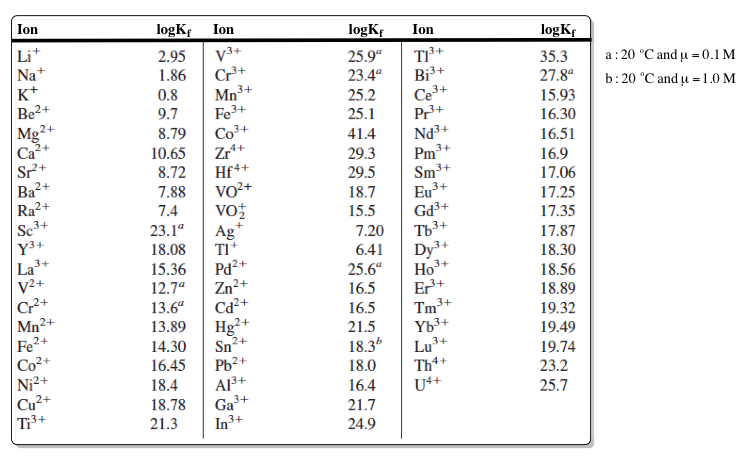The conditional formation constant can be calculated by the determining the fraction of the basic form and the formation constant of the metal ion.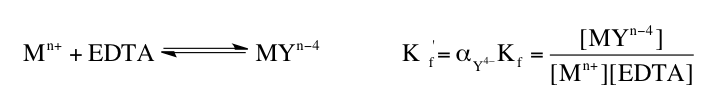6

#### example

EDTA Complexes7m
Play a video:

## EDTA Complexes Calculations

7

#### example

EDTA Complexes Calculations 13m
Play a video:
So for this question, it asked us to determine the conditional formation constant for this E. D. T. A complex at a ph of 8.0. Now remember our conditional formation constant is K prime sub F. It is equal to the fraction of the basic form of E. D. T. A. Which will designate his alpha times my formation or stability constant. Now here, if we take a look remember et itself is -4 in terms of its charge. When dealing with the basic form, the overall charge is minus one. For that to occur, Cobalt here would have to have a charge of plus three. So we're dealing with cobalt three ion interacting with E. D. T. A. To produce this E D. T. A complex. Now here they're telling us that our ph is 8.0. So remember in the chart that we saw a few pages ago when the ph is 8.0, then the fraction of the basic form of E D. T. A gives us a value of 4.2 times 10 to the minus three. Here, when we're dealing with whole integers in terms of ph so 0 to 14, we can simply look at that chart and pick the value that we see for the fraction of the basic form of E. D. T. A. Now remember if our ph is a value that is not a whole imager. So let's say we had uh 6.15 instead of 8.0, we have to utilize the equation that we've used before to figure out the fraction of the basic form. Remember that was the one where we had. K. A. One all the way to K. Six on top divided by H. Plus to the six plus all the other portion of portions of the denominator. So that one would be a longer process in order to figure out the the fraction of the basic form of R. E. D. T. A. Species. But again, we're dealing with whole numbers so we can simply look at the chart and pick the value that we see. Alright, so now we have that then when we're dealing with cobalt three while the log of K. F. For cobalt three ion equals 41.4. But we don't want the log of K. F. We just want K. F. So we're gonna take the inverse log function. So that's K. F. Of cobalt three ion equals 10 to 41.4. So we'll put 10-41.4 here And then multiply those two values together gives me an answer of 1.05 times 10 to the 39 as the conditional formation constant at that exact ph Now remember your K value is greater than one. That means that products are highly favored. Which would mean that when cobalt three ion comes into contact with E. T. T. A. There is a high propensity or high desire to form this complex here. So that's what our KF value was telling us here. And remember the more positive the charge of the metal ion, the greater the affinity the e d t, a molecule or Ligon has for the positive ion, the more they're gonna combine together to help make this complex here. Now that we've seen this simple example, move on to example to remember and utilize some of the techniques that we use in the previous page to help figure out the amount of free tend to ion within this example, given so, good luck guys.
8

#### example

EDTA Complexes Calculations 15m
Play a video: